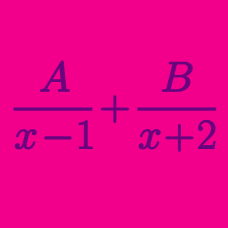Algebra

# Partial Fractions - Application to Integration

Evaluate $\int_6^{18}\frac{6x^2}{x^3+15x^2+72x+108}dx.$

What is $\int\frac{1}{x^2+7x+6}dx?$

Suppose that $$f(x)$$ is a function whose derivative is $f'(x)=\frac{1}{x^2-2x}$ and that $$f(-2)=\frac{1}{2}\ln2.$$ What is the value of $$f(8)?$$

What is $\int\frac{x+6}{x^2-9x+20}dx?$

What is $\int\frac{1}{x^2-16}dx?$

×

Problem Loading...

Note Loading...

Set Loading...